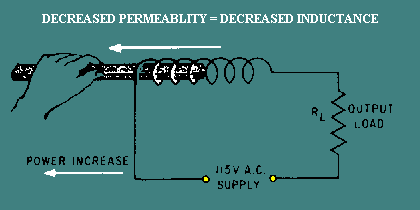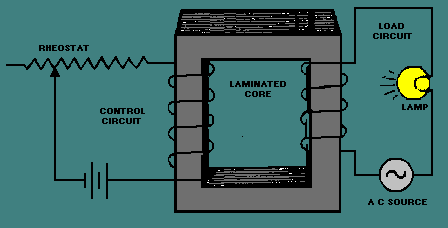inductance? Before answering that question, you should recall a few things about magnetism and inductors from NEETS, module 1 - Introduction to Matter, Energy, and Direct Current, chapter 1 - Matter, Energy, and Electricity; and module 2 - Introduction to Alternating Current and Transformers, chapter 2 - Inductance. ">Custom SearchSince changing the inductance of a coil enables the control of power to a load, what methods are available to change the inductance? Before answering that question, you should recall a few things about magnetism and inductors from NEETS, module 1 - Introduction to Matter, Energy, and Direct Current, chapter 1 - Matter, Energy, and Electricity; and module 2 - Introduction to Alternating Current and Transformers, chapter 2 - Inductance. Permeability was defined as the measure of the ability of a material to act as a path for additional magnetic lines of force. Soft iron was presented as having high permeability compared with air. In fact, the permeability of unmagnetized iron is 5000 while air has a permeability of 1. A nonmagnetized piece of iron has high permeability because the tiny molecular magnets (Weber's Theory) or the directions of electron spin (Domain Theory) are able to be aligned by a magnetic field. As they align, they act as a path for the magnetic lines of force. Earlier NEETS modules state that the inductance of a coil increases directly as the permeability of the core material increases. If a coil is wound around an iron core, the permeability of the core is 5000. Now, if the iron is pulled part way out of the coil of wire, the core is part iron and part air. The permeability of the core decreases. As the permeability of the core decreases, the inductance of the coil decreases. This increases the power delivered to the load (true power). This relationship is shown in figure 3-30. The system shown in figure 3-30 is not too practical. Even if a motor were used in place of the hand that is shown, the resulting amplifier would be large, expensive, and not easily controlled. If the permeability of a core could be changed by electrical means rather than mechanical, a more practical system would result. Figure 3-30. - Varying coil inductance with a movable coil.High permeability depends on there being many molecular magnets (or electron spin directions) that can be aligned to provide a path for magnetic lines of force. If almost all of these available paths are already being used, the material is magnetized and there are no more paths for additional lines of force. The "flux density" (number of lines of force passing through a given area) is as high as it can be. This means that the permeability of the material has decreased. When this condition is reached, the core is said to be SATURATED because it is saturated (filled) with all the magnetic lines of force it can pass. At this point, the core has almost the same value of permeability as air (1) instead of the much higher value of permeability (5000) that it had when it was unmagnetized. Of course, the permeability does not suddenly change from 5000 to 1. The permeability changes as the magnetizing force changes until saturation is reached. At saturation, permeability remains very low no matter how much the magnetizing force increases. If you were to draw a graph of the flux density compared to the magnetizing force, you would have something similar to the graph shown in figure 3-31. Figure 3-31 also includes a curve representing the value of permeability as the magnetizing force increases. Point "s" in figure 3-31 is the point of saturation. The flux density does not increase above point "s," and the permeability is at a steady, low value. Figure 3-31. - Magnetization and permeability curves.You have now seen how a change in the magnetizing force causes a change in permeability. The next question is, how do you change the magnetizing force? Magnetizing force is a function of AMPERE-TURNS. (An ampere-turn is the magnetomotive force developed by 1 ampere of current flowing in a coil of one turn.) If you increase the ampere-turns of a coil, the magnetizing force increases. Since it is not practical to increase the number of turns, the easiest way to accomplish this is to increase the current through the coil. If you increase the current through a coil, you increase the ampere-turns. By increasing the ampere-turns you increase the magnetizing force. At some point, this causes a decrease in the permeability of the core. With the permeability of the core decreased, the inductance of the coil decreases. As said before, a decrease in the inductance causes an increase in power through the load. A device that uses this arrangement is called a SATURABLE-CORE REACTOR or SATURABLE REACTOR. SATURABLE-CORE REACTOR A saturable-core reactor is a magnetic-core reactor (coil) whose reactance is controlled by changing the permeability of the core. The permeability of the core is changed by varying a unidirectional flux (flux in one direction) through the core. Figure 3-32 shows a saturable-core reactor that is used to control the intensity of a lamp. Notice that two coils are wound around a single core. The coil on the left is connected to a rheostat and a battery. This coil is called the control coil because it is part of the control circuit. The coil on the right is connected to a lamp (the load) and an a.c. source. This coil is called the load coil because it is part of the load circuit. As the wiper (the movable connection) of the rheostat is moved toward the right, there is less resistance in the control circuit. With less resistance, the control-circuit current increases. This causes the amount of magnetism in the core to increase and the inductance of the coil in the load circuit to decrease (because the core is common to both coils). With less inductance in the load circuit, load current increases and the lamp gets brighter. Figure 3-32. - A simple saturable-core reactor circuit.The schematic diagram of this circuit is shown in figure 3-33. L1 is the schematic symbol for a saturable-core reactor. The control winding is shown with five loops, and the load winding is shown with three loops. The double bar between the inductors stands for an iron core, and the symbol that cuts across the two windings is a saturable-core symbol indicating that the two windings share a saturable core. Figure 3-33. - Schematic diagram of a simple saturable-core reactor.Now that you have seen the basic operation of a saturable-core reactor, there is one other idea to discuss before moving on to the circuitry of a magnetic amplifier. There is a point upon the magnetization curve where the saturable-core reactor should be operated. The ideal operating point is the place in which a small increase in control current will cause a large increase in output power and a small decrease in control current will cause a large decrease in output power. This point is on the flattest portion of the permeability curve (after its peak). Figure 3-34 shows the magnetization and permeability curves for a saturable-core reactor with the ideal operating point (point "O") indicated. Notice point "O" on the magnetization curve. The portion of the magnetization curve where point "O" is located is called the KNEE OF THE CURVE. The knee of the curve is the point of maximum curvature. It is called the "knee" because it looks like the knee of a leg that is bent. Saturable-core reactors and magnetic amplifiers should be operated on the knee of the magnetization curve. Figure 3-34. - Magnetization and permeability curves with operating point.Integrated Publishing, Inc. - A (SDVOSB) Service Disabled Veteran Owned Small Business